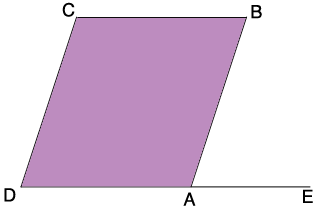SEARCH HOMEMath Central Quandaries & QueriesQuestion from Hanna, a student: The consecutive angles of a parallelogram measuresHi Hanna,

I drew a diagram of a parallelogram $ABCD$ and extended the side $DA$ to $E.$ The measure of the interior angle at $A$ is $x + 30$ and the measure of the interior angle at $B$ is $4x.$What do you know about the angle $BAE?$

PennyMath Central is supported by the University of Regina and the Imperial Oil Foundation.